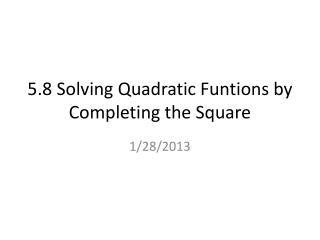DownloadDownload Presentation5.8 Solving Quadratic Funtions by Completing the Square

# 5.8 Solving Quadratic Funtions by Completing the Square

Télécharger la présentation## 5.8 Solving Quadratic Funtions by Completing the Square

- - - - - - - - - - - - - - - - - - - - - - - - - - - E N D - - - - - - - - - - - - - - - - - - - - - - - - - - -
##### Presentation Transcript

1. Vocabulary Whenever you multiply a binomial by itself, the resulting trinomial is called a perfect square trinomial Perfect Square Trinomial: Example: This is the area of this square x+1 x+1

2. Vocabulary The process of adding a constant c to the expression x2 + bx to make it a perfect square trinomial (PST) Completing the Square : How? By adding to x2 + bx What is it used for: Converting equations from standard form to vertex form. To solve quadratic functions when “Big X” does not work! Factored form of PST: The square of 2 binomials

3. Review Equation of a Parabola in VERTEX FORM: Where (h, k) is the vertex

4. Steps for completing the square Standard form : y = x2 + bx + c y = x2 + bx + c ( ) This is a PST y = (x2 + bx+ + c - Factored form of PST: • Put ( ) around x2 + bx and move c outside ( ) • Take half of b and square it. Add it to the ( ) and subtract it from c. • 3. Factor the PST in ( ) and simplify c - y = (x + 2 + d Note: if y = x2–bx + c Then y = (x -)2 + d

5. Example 1 Rewrite y = x2 + 6x + 5 in Vertex Form and determine the vertex. y = x2 + 6x ( ) + 5 • Put ( ) around x2 + 6x and move +5 outside ( ) • Take half of 6 and square it. Add 9 to the ( ) and subtract 9 from 5. • 3. Factor the PST in ( ) and simplify This is a PST y = (x2 + 6x + + 5 - Factored form of PST: y = (x + 2 - 4 Vertex (-3, -4)

6. Example 2 Rewrite y = x2- 6x + 10 in Vertex Form and determine the vertex. y = x2- 6x ( ) + 10 • Put ( ) around x2 - 6x and move +10 outside ( ) • Take half of 6 and square it. Add 9 to the ( ) and subtract 9 from 10. • 3. Rewrite what’s in the ( ) as (x - 3)2 y = (x2- 6x + + 10 - y = (x -2 + 1 Vertex (3, 1)

7. Checkpoint ANSWER ( )2 – + y 3; x 4 = ( ) 4, 3 – y x2 8x + 19 = Use Completing the Square Write in vertex form. Then identify the vertex.

8. Homework: 5.8 p.271 #15-20